Cai, H. (2019). "Wetting behavior and surface energy of bamboo fiber determined via dynamic contact angle analysis using the Wilhelmy technique," BioRes. 14(3), 5121-5131.

#### Abstract

The object of this paper was to obtain stable and reproducible dynamic contact angles (CA) and calculate the surface energy (SE) of bamboo fiber. The average wetted perimeter of bamboo fiber was determined using the Wilhelmy technique. The dispersion and polar components of the bamboo fiber at different immersion velocities were also investigated using the Owens-Wendt approach. The results indicated that the use of the mean wetted perimeter of 30 fibers to estimate the dynamic contact angle was feasible and accurate. As the measured velocity increased, the dynamic advancing contact angle of bamboo fiber first rapidly increased and then gradually stabilized. The surface energy of bamboo fiber presented a tendency of nonlinear decrease when the test speed increased. A measured velocity set from 1 mm/min to 3 mm/min was reasonable for the bamboo fiber dynamic CA test, with an obtained SE of 41.7 mN/m to 43.6 mN/m. A correlation and partial correlation analysis showed that the reliability coefficient had 14.1% correlation between the dynamic CA and measure velocity was induced by the synergistic effect of the testing liquid. The SE of bamboo fiber was negatively correlated to its dynamic advancing CA.

Wetting Behavior and Surface Energy of Bamboo Fiber Determined via Dynamic Contact Angle Analysis Using the Wilhelmy Technique

Hanzhong Cai *

The object of this paper was to obtain stable and reproducible dynamic contact angles (CA) and calculate the surface energy (SE) of bamboo fiber. The average wetted perimeter of bamboo fiber was determined using the Wilhelmy technique. The dispersion and polar components of the bamboo fiber at different immersion velocities were also investigated using the Owens-Wendt approach. The results indicated that the use of the mean wetted perimeter of 30 fibers to estimate the dynamic contact angle was feasible and accurate. As the measured velocity increased, the dynamic advancing contact angle of bamboo fiber first rapidly increased and then gradually stabilized. The surface energy of bamboo fiber presented a tendency of nonlinear decrease when the test speed increased. A measured velocity set from 1 mm/min to 3 mm/min was reasonable for the bamboo fiber dynamic CA test, with an obtained SE of 41.7 mN/m to 43.6 mN/m. A correlation and partial correlation analysis showed that the reliability coefficient had 14.1% correlation between the dynamic CA and measure velocity was induced by the synergistic effect of the testing liquid. The SE of bamboo fiber was negatively correlated to its dynamic advancing CA.

Keywords: Bamboo fiber; Wetting perimeter; Dynamic contact angle; Measure speed; Surface energy

Contact information: Key Laboratory of Wood Material Science and Engineering of Jilin Province, Beihua University, Jilin, Jilin Province, China 132013; *Corresponding author: Zxcchz20050622@163.com

INTRODUCTION

Bamboo fiber possesses properties between that of grass and wood and has attracted great interest as a sustainable environmental reinforcement in composite materials due to its excellent mechanical properties, high abundance, fast-growing speed, and low cost (Chen et al. 2016; Sun et al. 2018). Its specific strength and specific modulus are comparable to those of glass fiber (Tran et al. 2011). These characteristics give bamboo fiber potential to manufacture high-performance green composites (Nugroho and Ando 2001; Tian et al. 2019).

The interphase property of composites between the fiber and resin matrix has an important effect on the final mechanical performance, because the bonding strength, stress transfer, and load distribution efficiency at the interface is determined by the degree of the interfacial interactions, such as physical adhesion, chemical bonding, and mechanical interlocking (Defoirdt et al. 2010; Fuentes et al. 2011). A more even and higher quality of physical adhesion results in better wetting between the fibers and resin obtained, which is controlled by the surface energy of the bamboo fiber. The surface energy of fibers can be examined by studying its wetting behaviors with different probe liquids. The fibers and matrices can then be reasonably matched in accordance to the dispersion and polar components of their surface energy, which provides a method to improve the interfacial compatibility and performance (Rebouillat et al. 1999; Espert et al. 2004). Therefore, the surface energy of the fiber and the compatibility between the fiber and resin is verified and predicted using dynamical contact angle experimental data.

Currently, there are two very common methods of obtaining the contact angle. Both quantitatively measure the solid-liquid interactions in terms of the equilibrium contact angle, following the definition of Young (Young 1805; Le et al. 1996; Seveno and De Coninck 2004). The first one is called the optical method and is a direct technique of introducing a drop of liquid on the fiber and then analyzing the fluid geometry change during the wetting procedure. The second method is the Wilhelmy technique, which is an indirect method that uses a microbalance to record the data of wetting force variation when the liquid spread upwards on the fiber. The recorded data are further evaluated with respect to the wetting perimeter of the fiber and surface tension of the testing liquid.

Compared with synthetic fibers, additional complexities of wetting behavior have been found for the natural plant fibers that may affect the measurement results of contact angle and surface energy. These complexities include liquid sorption/diffusion into the surface layer or cells, diffusion of low-molecular-weight compounds from the surface layers into the liquid, inhomogeneity of density distribution and chemical constituents of the fiber surface layers or cells, and viscoelastic response of the surface layers to the liquid (Silva et al. 1999; Lancet 2003).

Additionally, bamboo is a typical functionally graded biomaterial, its vascular bundle of parenchymal cells is regularly distributed along the radius direction (Zhang et al. 2014; Sharma et al. 2015). A multilayer hierarchical microstructure of bamboo fiber is observed with a thick layer and thin layer alternate arrangement on the cell wall (Fig. 1). The complexity, as mentioned above, brings great challenges to the characterization of wetting behaviors for bamboo fibers.

In this paper, the average wetting perimeters of bamboo fibers were studied using the Wilhelmy technique and then their dynamic contact angles were examined with different immersion speeds and probe liquids. The polar and dispersion components of the surface energy of bamboo fiber were then estimated following the Owens-Wendt approach. Finally, the relationship between the measure speed and dynamic contact angle, as well as between the measure speed and the surface energy were analyzed. The object of the present study was to obtain a deep understanding of bamboo fiber’s wetting behaviors and surface energy.

EXPERIMENTAL

Materials

Sample preparation

Cizhu bamboo (Neosinocalamus affinis) fibers with a length of 50 to 120 mm were obtained via mechanical carding technology produced by Huasheng Zhuzhou Cedar Limited Company, Hunan province, China. Glass fibers were provided by Ningbo Shuaibang Chemical Fibre, Co., Ltd., Zhejiang province, China. Bamboo fibers with a uniform diameter and straight morphology were picked out using precision tweezers and then soaked in 70 °C water for 1 h. After removing the surface grease with ethanol, the fibers were rinsed with the deionized water twice and were then oven-dried at 65 °C for 1 h. Finally, the fibers were placed in the constant temperature and humidity box at 20 °C and 65% relative humidity for at least 48 h.

Test equipment and liquid

A K100 surface tension instrument (KRÜSS GmbH, Hamburg, Germany) with an accuracy of 1 µg was used in this study. The instrument has the following advantages: 1) The ion wind device can remove static electricity on the surface of the fibers; 2) The test parameters can be automatically set, including water depth into the fiber, sensitivity, test speed, and data collection frequency; and 3) The total time of wetting force variation can be controlled to keep the test stability of the operation elevator. The parameters of the liquid used for bamboo fiber wetting test are shown in Table 1.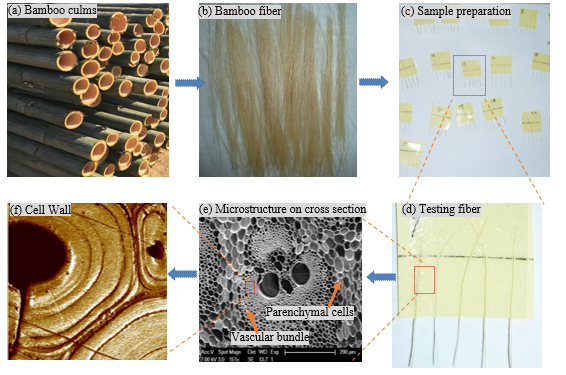Fig. 1. Preparation of bamboo wetting test samples and its microstructure

Table 1. Parameters of the Test Liquids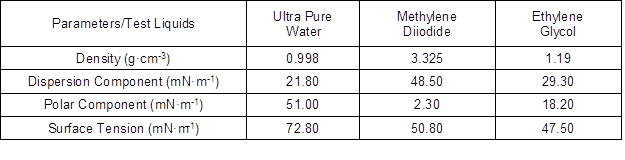Methods

Measurement of wetted perimeter

Method 1: The average diameters (D) at the 5 mm distance to the end of fibers were measured using the Leica integral microscope (Leica Microsystems Inc., Wetzlar, Germany), and then the wetted perimeters (P) could be calculated using Eq. 1: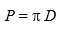(1)

Method 2: The one end of the fibers tested was vertically immersed into n-hexane, and the other end was clamped by the chuck fixture of the K100 surface tension instrument. The wetting depth, wetting velocity, and the sensitivity were set at 5 mm, 3 mm/min, and 0.0001 g, respectively. The total measuring force of the balance (Ftotal) was calculated using the Wilhelmy Formula, or Eq. 2,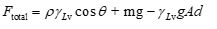(2)

where p is the wetted perimeter of the fiber (mm), rlv is the surface tension of the liquid (mN·m-1), θ is contact angle (°), m is the fiber quality, g is the gravity acceleration (N/g), ρis the liquid density (g/cm3), A is the cross-section area (mm2) of the fibers, and d is the infiltration depth (mm).

Before the liquid’s contact with the fiber, the weight was auto-set to zero. To calculate the wetting force, the buoyancy will be deducted when the wetting depth is zero. Then, the modified Wilhelmy equation was as follows in Eq. 3,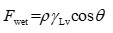(3)

where Fwet is the wetting force (µN) after automatic correction.

The surface tension of the n-hexane is very small, so the fiber was completely wetted and the contact angle was 0° under a relatively low wetting speed. The following Eq. 4 was obtained,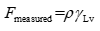(4)

where Fmeasured is the complete wetting force (µN) after automatic correction. The wetted perimeters of the fibers were calculated using Eq. 4 and the test results are shown in Fig. 3.

Measurement of dynamic contact angle

The wetting velocities were set as 0.15, 0.5, 1, 3, 10, 60, 100, 200, 300, 400, and 500 mm/min. At each test velocity there were 6 groups that each included 5 fibers, for a total of 990 fibers that were respectively tested in three kinds of testing liquid. The immersion depth was 5 mm, the ambient temperature and relative humidity were 23 ± 2 °C and 60 ± 5%, respectively.

Estimation of surface free energy

Following the Owens-Wendt approach, surface free energy (rsv) is composed of the dispersion component (rdsv) and polar component (rpsv):

rsvrdsvrps (5)

The interfacial energy (rLv) between two phases was obtained using the following equation: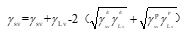(6)

Simultaneous Young equation: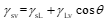(7)

To get: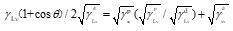(8)

Equation 9 can be converted to a linear equation,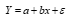(9)

where Y indicates x =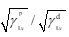，a =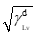，b =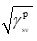, and ε is an error term, the square of the intercept and slope of the Eq. 9 is the dispersion component and the polar component.

Consistency inspection of marginal subset of dynamic contact angle at different immersion velocities by the Tukey HSD using the software IBW SPSS Statistics 21.

RESULTS AND DISCUSSION

Measurement of Wetted Perimeter and Dynamic Contact Angle of Fibers

As shown in Fig. 2(a), the curves of the wetting force-position for bamboo fiber greatly fluctuated compared to that of glass fiber. Bamboo fiber as a plant fiber is composed of an inhomogeneous distribution of chemical constituents (cellulose, hemicellulose, and lignin), which resulted in the uneven distribution of hydrophilic groups in the fibers. Meanwhile, multilayer structures of the cell wall (Figs. 1(e) and 1(f)), and large porosity on the fiber’s cross-section were the main reasons that the wetting load-position curve notably fluctuated. The glass fiber is a type of chemical fiber with a smooth surface, uniform composition, and compact structure.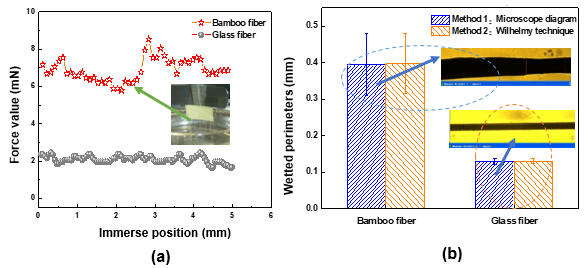Fig. 2. Wetted perimeters measurement of bamboo fiber and glass fiber: (a) Wetting force-position curves or (b) Comparison of wetted perimeter measurements by two methods

It was very difficult to measure and evaluate the bamboo fiber’s wetting perimeter and dynamic contact angles due to its uneven thickness and variability (Fuentes et al. 2011). A low relative error of the wetting perimeter is shown in Fig. 2(b), which was measured using the n-hexane mechanical method (Method 2, in Fig. 2(b)) and image analysis method (Method 1, in Fig. 2(b)). The relative errors for the wetting perimeter of glass fiber and bamboo fiber were 1.42% and 3.69%, respectively, and the relative error ranges were 0.5% to 3% and 0.44% to 9.7%, respectively. It could be seen that the n-hexane method could be used to accurately and rapidly evaluate the average wetting perimeter of variant plant fibers in a certain length range.

The fiber’s wetting perimeter was first calculated according to the linear fitting equation (Fig. 3) from Eq. 4, and then the advancing contact angles and the receding contact angles were obtained by Eq. 3. When the wetting force greatly changed, the dynamic advancing contact angles of fiber noticeably changed. The dynamic receding CA was always 0° because the fiber was influenced by the liquid in the immersion process. Therefore, the dynamic advanced contact angles were used to characterize the fibers wettability herein.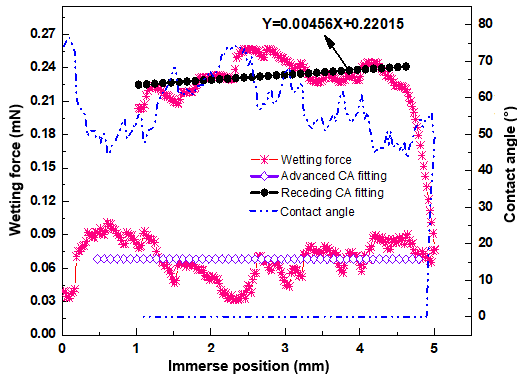Fig. 3. Dynamic Wetting curves of bamboo fiber in the water

Table 2. Contact Angle Calculated by Different Measurements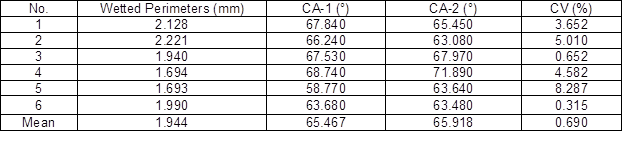Note: Wetted perimeters here are five bamboo fibers, CA-1 is the contact angle of the wetted perimeters calculated by the image analysis method (Method 2 in Fig. 2(b)), CA-2 is the contact angle of the average wetted perimeters of 30 bamboo fibers by using the n-hexane method (Method 1 in Fig. 2(b))

As shown in Table 2, the relative variation of the fiber contact angles evaluated by the two different methods was low, with an average difference value of 0.45° and mean variance (0.69%) of less than 1%. Therefore, it is feasible for the plant fiber that has natural variability to measure the average wetted perimeter and CA via the n-hexane method (Method 1, in Fig. 2(b)).

Effect of Wetting Velocity on Dynamic Contact Angles

As shown in Fig. 4, with an increased testing rate, the dynamic contact angle of bamboo fibers rapidly increased and then gradually stabilized in all of the tested liquids. This finding was consistent with the simulation trend of the molecular dynamics model (Tran et al. 2011), as shown in Fig. 4,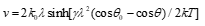(10)

where v is the test rate and k0 is the equilibrium displacement frequency (mm/min), sinh is the hyperbolic function, γ is the liquid surface tension (mN·m-1), k is the Boltzmann constant, and θ and θ0 present the forward dynamic contact angles and the equilibrium dynamic contact angles (°), respectively.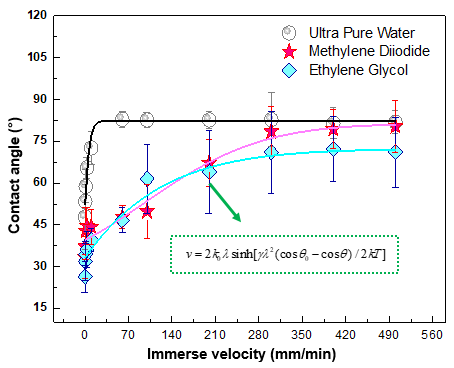Fig. 4. Dynamic contact angle of bamboo fiber at different immersion velocities

The marginal mean can predict the magnitude of the interaction between the dependent variable and the factor variable at each level of the factor variable. As shown in Table 3, the marginal mean of the advanced dynamic contact angle at 11 immersion velocity levels was divided into seven subsets. No obvious difference between the marginal mean of each subset was found (p > 0.05). At the low velocity of [0.15, 10] tests: 0.15 to 1 mm/min, 0.5 to 3 mm/min, and 3 to 10 mm/min, each of the three immersion velocities belonged to the same subset, respectively. At the middle test rate of [10, 200]: 10 to 60 mm/min, 60 to 100 mm/min, and 100 to 200 mm/min, each of the two test rates belonged to the same subset, respectively. At the high velocity of [200, 500] tests: each velocity of 200 mm/min, 300 mm/min, 400 mm/min, and 500 mm/min belonged to a separate subset. The dynamic CA evaluated at the high immersion velocity was higher than that at the low velocity. This was consistent with the analysis of variance; that is, the main effect of the model was a highly significant difference (p < 0.001, alpha = 0.01).

The contact angle not only related to material property tested, but also to the testing speeds. The correlation analysis between the contact angle and immersion velocity of bamboo fibers showed that Pearson’s correlation coefficient was 0.749 (P < 0.001), and the partial correlation coefficient was 0.8379 (P < 0.001) when the control of testing method. The correlation degree of 14.11% between the dynamic CA and immersion velocity was produced via the synergistic effect of the test liquid.

Table 3. Consistency Inspection of Marginal Subset of Dynamic Contact Angle at Different Immersion Velocities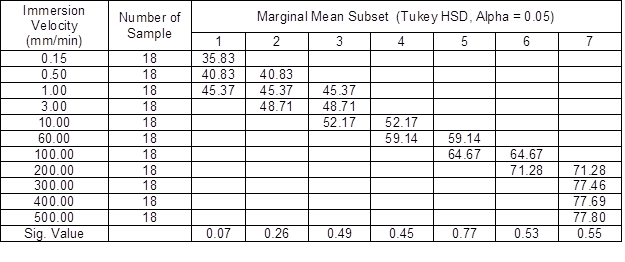Characterization of Surface Energy of Bamboo Fiber

Table 4 shows the polar and dispersion components of the surface energy of bamboo fibers at different measure speeds. Figure 5 was the nonlinear fitting curves of surface energy vs.measure speeds of bamboo fiber and its first-order differential curve.

It was found that the apparent dispersion component of bamboo fiber first increased and then decreased as the measure speed increased, while the apparent polar component presented an opposite trend. When the measure speed increased to 300 mm/min, both were stable at 11 to 12 mN/m. However, the value of surface energy of bamboo fiber decreased nonlinearly with an increase in measure speed, as shown in Fig. 5.

A higher measure speed setting resulted in a shorter time for bamboo fiber contact with the test liquid, and the fibers had a low probability to be fully wetted by the liquid; thus it presented a low wetting force. The contact angle in the range of 0 to 180° was inversely proportional to the wetting force via Eq. 3. Hence, a higher contact angle would be obtained at a high measure speed. According to Eq. 7, if the interfacial energy was an invariable situation, the apparent surface energy of material decreased as the contact angle increased.

When the measure speed was lower than 1 mm/min, the range of the fiber surface energy was [43.61, 49.97] mN/m, with a variation of [-7.41, -4.39] mN/m by the first-order differential curve. When the test rate was more than 3 mm/min, the change rate of the surface energy tended to be 0 mN/m. Therefore, it was reasonable that the test rate was set at 1 to 3 mm/min, with a 41.71 to 43.61 mN/m surface energy, which was almost consistent with the reference (Chen et al. 2004; Lu et al. 2012).

The dispersion component and the polar component of the wax were 26.5 mN/m and 0 mN/m, respectively (Maximova et al. 2004; Tran et al. 2011), and those of the lignin were 52.5 mN/m and 40 to 43.5mN/m, respectively. The surface energy of bamboo fiber was greater than that of wax, but lower than that of the lignin. It was indicated that the surface of bamboo fiber not only possessed a hydrophobic non-polar component, but also its own hydrophilic polar groups, which resulted in the complex wetting characteristics.

Table 4. Dispersion and Polar Components of Surface Energy of Bamboo Fiber at Different Measure Speeds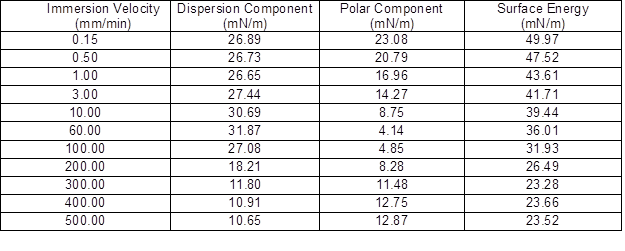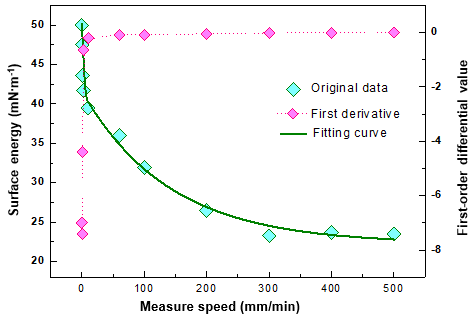Fig. 5. Nonlinear fitting curves of surface energy vs. measure speeds and its first derivative

CONCLUSIONS

1. The method of estimating the dynamic advance contact angle of bamboo fibers by calculating the average wetting perimeter of bamboo fibers with the n-hexane method was more convenient and accurate than the traditional image measurement method.
2. With increased test speeds, the dynamic advancing contact angle was consistent with the molecular dynamics model, namely first a rapid increase and then gradual stability.
3. Bamboo fiber wetting behavior presented both none-hydrophobic and polar properties, with a surface energy of 41.7 to 43.6 mN/m. The relationship between the calculated surface energy of bamboo fiber and the dynamic advance contact angle was negatively linear.
4. Quantitative characterization of the surface energy and chemical composition provide important references for the optimization of interfacial properties and the improvement of mechanical strength as well as durability of bamboo fiber-reinforced composites.

ACKNOWLEDGEMENTS

The authors are grateful for the constructive comments from the anonymous reviewers that are greatly appreciated.

REFERENCES CITED

Chen, F., Jiang, Z., Wang, G., Li, H. D., Smith, L. M., and Shi, S. Q. (2016). “The bending properties of bamboo bundle laminated veneer lumber (BLVL) double beams,” Construction and Building Materials 119, 145-151. DOI: 10.1016/j.conbuildmat.2016.03.114

Chen, Y., Gao, Y., and Shen, Q. (2004). “Comparisons of the surface properties of bamboo fiber and a comparison with cotton linter fiber,” Journal of Cellulose Science and Technology12(2), 6-9. DOI: 10.3969/j.issn.1004-8405.2004.02.002

Defoirdt, N., Biswas, S., De Vries, L., Tran, L., Van Acker, J., Ahsan, Q., Gorbatikh, L., Van Vuure, A., and Verpoest, I. (2010). “Assessment of the tensile properties of coir, bamboo and jute fibre,” Composites Part A: Applied Science and Manufacturing 41(5), 588-595. DOI: 10.1016/ j.compositesa.2010.01.005

Espert, A., Vilaplana, F., and Karlsson, S. (2004). “Comparison of water absorption in natural cellulosic fibres from wood and one-year crops in polypropylene composites and its influence on their mechanical properties,” Composites Part A: Applied Science and Manufacturing 35(11), 1267-1276. DOI: 10.1016/j.compositesa.2004.04.004

Fuentes, C., Tran, L., Dupont-Gillain, C., Vanderlinden, W., Feyter, S., Vuure, A., and Verpoest, I. (2011). “Wetting behavior and surface properties of technical bamboo fibres,” Colloids and Surfaces A: Physicochemical and Engineering Aspects 380(1-3), 89-99. DOI: 10.1016/ j. colsurfa.2011.02.032

Lancet, T. (2003). “Wetting behavior of flax fibers as reinforcement for polypropylene,” Journal of Colloid and Interface Science 263(2), 580-589. DOI: 10.1016/S0021-9797(03)00294-7

Le, C., Ly, N., and Stevens, G. (1996). “Measuring the contact angles of liquid droplets on wool fibers and determining surface energy component,” Textile Research Journal 66(6), 389-397. DOI: 10.1177/ 004051759606600606

Lu, J., Zhang, H., Wei, D., and Hu, Y. (2012). “A method for determining surface free energy of bamboo fiber materials by applying Fowkes theory and using computer aided machine vision based measurement technique,” Journal of Shanghai Jiaotong University (Science) 17(5), 593-597. DOI: 10.1007/s12204-012-1330-9

Maximova, N., Sterberg, M., Laine, J., and Stenius, P. (2004). “The wetting properties and morphology of lignin adsorbed on cellulose fibres and mica,” Colloids and Surfaces A: Physicochemical and Engineering Aspects 239(1-3), 65-75. DOI: 10.1016/j.colsurfa.2004.01.015

Nugroho, N., and Ando, N. (2001). “Development of structural composite products made from bamboo II: Fundamental properties of laminated bamboo lumber,” Journal of Wood Science 47(3), 237-242. DOI: 10.1007/BF01171228

Rebouillat, S., Letellier, B., and Steffenino, B. (1999). “Wettability of single fibres – beyond the contact angle approach,” International Journal of Adhesion and Adhesives 19(4), 303-314. DOI: 10.1016/S0143-7496(99)00006-8

Seveno, D., and De Coninck, J. (2004). “Possibility of different time scales in the capillary rise around a fiber,” Langmuir 20(3), 737-742. DOI: 10.1021/la030263h

Sharma, B., Gatόo, A., Bock, M., and Ramage, M. (2015). “Engineered bamboo for structural applications,” Construction and Building Materials 81, 66-73. DOI: 10.1016/ j. conbuildmat. 2015. 01.077

Sun, Y., Jiang, Z., Zhang, X., Sun, Z., Yang, X., and Liu, H. (2018). “The impact performance of bamboo oriented strand board and computed tomography technique for detecting internal damage,” BioResources 13(3), 6707-6721. DOI: 10.15376/biores.13.3.6707-6721

Tian, L., Kou, Y., and Hao, J. P. (2019). “Flexural behavior of sprayed lightweight composite mortar-original bamboo composite beams: experimental study,” BioResources 14(1), 500-517. DOI: 10.15376 /biores.14.1.500-517

Tran, L., Fuentes, C., Dupont-Gillain, C., Vuure, A., and Verpoest, I. (2011). “Wetting analysis and surface characterization of coir fibres used as reinforcement for composites,” Colloids and Surfaces A: Physicochemical and Engineering Aspects 377(1-3), 251-260. DOI: 10.1016/ j.colsurfa. 2011.01.023

Young, T. (1805). “An essay on the cohesion of fluids,” Philosophical Transactions of the Royal Society of London 95, 65-87, DOI:10.1098/rstl.1805.0005

Zhang, D., Wang, G., and Ren, W. (2014). “Effect of different veneer-joint forms and allocations on mechanical properties of bamboo-bundle laminated veneer lumber,” BioResources 9(2), 2689-2695. DOI: 10.15376/biores.9.2.2689-2695

Article submitted: January 27, 2019; Peer review completed: May 4, 2019; Revised version received: May 7, 2019; Accepted: May 8, 2019; Published: May 9, 2019.

DOI: 10.15376/biores.14.3.5121-5131